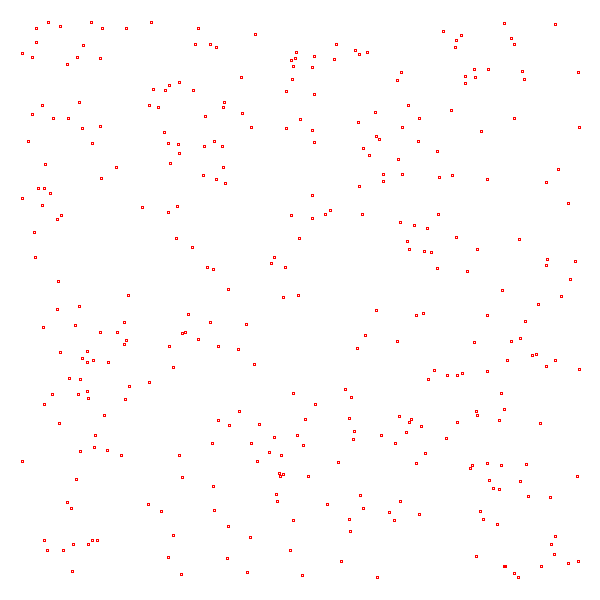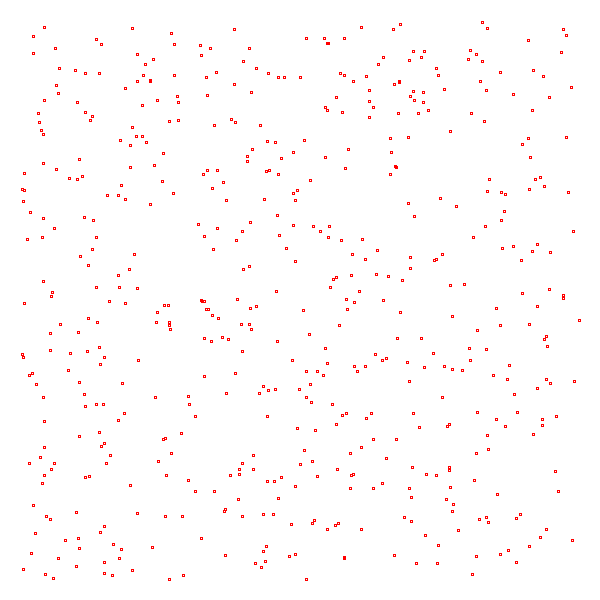JOINProblem Statement
Contest: Marathon Match 56
Problem: EnclosingCircles

### Problem Statement

You are given N points on a two-dimensional plane. You have to enclose these points with at most M circles so that each point is inside of at least one circle. Your task is to minimize the sum of areas of the circles you use.

### Implementation Details

Your code should implement one method placeCircles. int[]s pointX and pointY give you the coordinates of the points on the plane, and M is the maximal number of circles you can use.

You must return the list of the circles you'll use to enclose these points. Each element of your return describes one circle and should be formatted as "CENTERX CENTERY RADIUS" (quotes for clarity only). CENTERX and CENTERY specify x- and y-coordinates of the circle's center, and RADIUS specifies its radius. RADIUS must be strictly greater than 0.1. All values are double precision.

### Scoring

Your score for an individual test case will be the sum of the areas of the circles you used. Note that the score is NOT equal to the area on the plane covered with the circles; if the returned circles overlap, the area of the overlap is counted for each overlapping circle. If at least one of the given points is outside of all circles, your score for this test case will be zero. An invalid return of any kind will result in zero absolute score for that test case.

Your overall score will be the sum over all test cases of max(0, 400000 - AREA) / 1000, where AREA is the area you used.

### Test Case Generation

The number of points N is chosen between 50 and 1000, inclusive.

The maximal number of circles M is chosen between 10 and max(10, floor(N / 10)), inclusive.

x- and y-coordinates of each point are chosen between 0 and 511, inclusive.

You will have the opportunity to submit four test cases during the first week of the contest. Of the four, one will be selected at random for provisional testing, while the others will be used for final testing. After the first week, the submitted test cases will be locked. Your code should implement a method generateTestCase that takes one int argument and returns a int[] that contains the information about the test case that you want to submit. The first element must contain M. The second element must contain N. The next N elements should contain the values for pointX, following that another N elements that contain the values for pointY. This solution will be called four times on some tests -- you should not count on it being called every time. The test case will be validated and only valid test cases will be accepted.

### Visualizer

A visualizer is available for offline testing.

### Definition

 Class: EnclosingCircles Method: placeCircles Parameters: int[], int[], int Returns: String[] Method signature: String[] placeCircles(int[] pointX, int[] pointY, int M) Method: generateTestCase Parameters: int Returns: int[] Method signature: int[] generateTestCase(int testNumber) (be sure your methods are public)

### Notes

-All variables are chosen randomly, independently and uniformly.
-For more implementation details see the visualizer source.
-The memory limit is 1024 MB and the time limit for placeCircles() is 20 seconds (which includes only time spent in your code). The time limit for generateTestCase() is 5 seconds per call.
-There are 10 example test cases and 200 full submission test cases. The number of test cases will remain constant. The user generated tests will replace the randomly generated ones over the course of the first week.
-A point (px, py) is considered to be inside a circle (cx, cy) with radius (R) if ((cx-px)*(cx-px) + (cy-py)*(cy-py)) <= R*R.
-Your submission will only be tested against the test suite at the time that you submit it. This means that as new tests arrive, the scores may become somewhat stale.
-You must implement the generateTestCase() method, but is not obliged to return a valid case. Returning an empty array is ok, but we encourage you to generate some test cases.

### Examples

0)

```seed = 1
N = 321
M = 17
```1)

```seed = 2
N = 344
M = 25
```2)

```seed = 3
N = 558
M = 54
```3)

 ```seed = 4 N = 170 M = 13 ```
4)

 ```seed = 5 N = 205 M = 20 ```
5)

 ```seed = 6 N = 872 M = 49 ```
6)

 ```seed = 7 N = 509 M = 28 ```
7)

 ```seed = 8 N = 165 M = 16 ```
8)

 ```seed = 9 N = 219 M = 19 ```
9)

 ```seed = 10 N = 396 M = 20 ```

This problem statement is the exclusive and proprietary property of TopCoder, Inc. Any unauthorized use or reproduction of this information without the prior written consent of TopCoder, Inc. is strictly prohibited. (c)2010, TopCoder, Inc. All rights reserved.